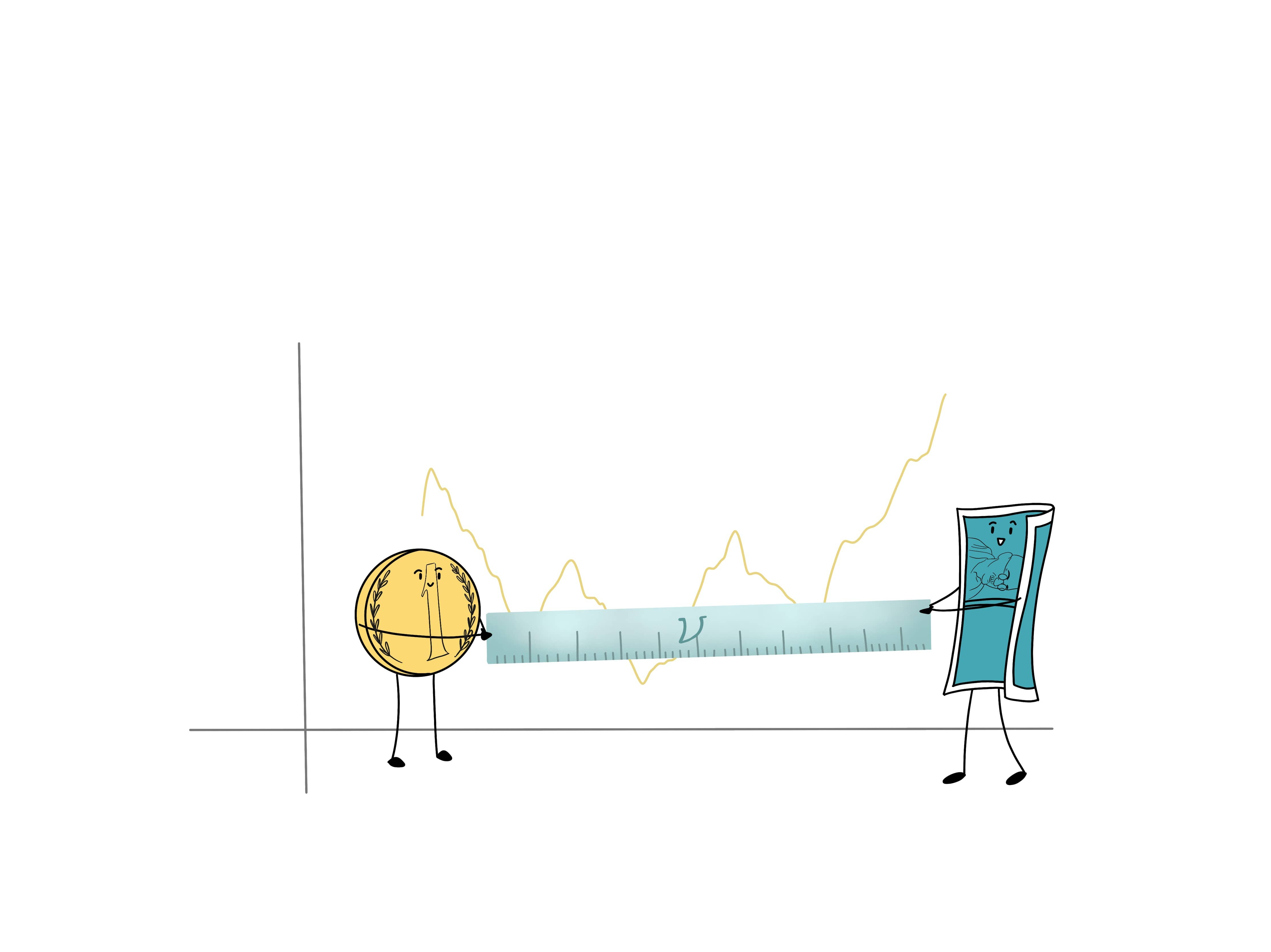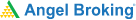# The greek of volatality: Vega## What is Vega?

• Vega is one of the Options Greeks that helps measure the risk of change in implied volatility.
• When the volatility of an asset increases, the price of the corresponding option also increases, since it is more likely for the asset to hit the strike price.
• The more (or less) the option price changes to a 1% change in implied volatility of the underlying asset, the greater (or smaller) the vega of the option is.
• Depending on the position, Vega can either be positive or negative.
• Long positions in options come with positive vega, whereas, short positions in options come with negative vega. This is regardless of whether the option is a call or put.
• When option price rises, traders in long positions benefit, while the ones in short positions lose if the option is exercised.
• Vega is not linear, and it can be affected by several factors.

### Characteristics of Vega

There are two factors that affect vega: moneyness and the amount of time left until the expiration date.

Vega is at its highest when an options contract is at-the-money. It reduces if the contract moves into-the-money or out-of-the-money. Remember, the further away the price of the underlying security is from the strike price, the lower is the vega value of that contract. As the extrinsic value of an option tends to be higher the closer it is to the money, and the vega value only affects extrinsic value, it stands to reason that this would be the case.

Similarly, the vega value will be higher when the time to expiration is far away, and lower when the time to expiration is near. You’d be surprised to know that Vega is also closely related to Gamma and is affected by it. When the gamma value of an option is high, you can expect the vega value to also be high.

### Putting Vega to Use

Usually, it is the delta, theta and gamma options that receive the most attention. This could probably be because vega is more complex to understand; it requires an in-depth understanding of volatility and implied volatility. However, after delta, it is vega that can be extremely useful in forecasting how the price of an option will change.

Various trading strategies can be used to profit from changes in volatility, even when the price of the underlying asset remains static. When such strategies are used, you will need If you wish to use such strategies, such as the long straddle or the short straddle, then the knowledge of Vega is of utmost importance.

Learning & Earning is now super simple₹ 0 Equity Delivery

No Hidden Charges₹ 20 Per Order For Intraday

FAQ,Currencies & CommoditiesZERO Brokerage*

on ALL SegmentsFREE Margin

Trade Funding

### Implied Volatility

Implied volatility is calculated using an option pricing model that determines what the current market prices are estimating an underlying asset's future volatility to be. Vega measures the theoretical price change for each percentage point move in implied volatility. Just like price moves, vega is also not always uniform; it changes over time. Hence, vega needs to be monitored regularly.

#### Wrapping up

An Example of Using Vega

Let’s assume that stock ABC is trading at Rs 450 in May. A June 50 call option on the same stock is selling for Rs 50, which is the premium. A June 50 call simply means that the option is a call option, with a Rs 500 strike price that expires in June.

For the purpose of this example, let’s assume that the implied volatility is 14%, and the vega of the option is 0.15. If the implied volatility increased to 15%, then the price of the June 50 call option would increase to Rs 50.15 (50 + 0.15).

If the implied volatility had instead decreased to 13%, then the price of the option would decline to Rs 49.85 (50 – 0.15).

#### A quick recap

• Vega helps measure the risk of change in implied volatility.
• Vega can either be positive or negative.
• Vega is not linear, and it can be affected by several factors.
• The two factors that affect vega are moneyness and time left to expiration.
• Vega is at its highest when an options contract is at-the-money. It reduces if the contract moves into-the-money or out-of-the-money.
• Similarly, the vega value will be higher when the time to expiration is far away, and lower when the time to expiration is near.Test Your Knowledge

Take the quiz for this chapter & mark it complete.

Comments (1)

Chavi Goel

08 May 2021, 05:25 PM

The quiz questions when is vega the highest and as per the module it is suppose to be at ATM, whereas the answer in the quiz is ITM similarly for the module there were 2 wrong answers. Kindly Take a look.

Thank You

Replies (1)
Add Comment

Get Information Mindfulness!

Catch-up With Market

News in 60 Seconds.The perfect starter to begin and stay tuned with your learning journey anytime and anywhere.Ready To Trade? Start withOpen an account